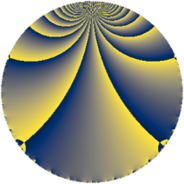# Properties

 Label 784.4.uLevel $784$ Weight $4$ Character orbit 784.u Rep. character $\chi_{784}(113,\cdot)$ Character field $\Q(\zeta_{7})$ Dimension $498$ Sturm bound $448$

# Related objects

## Defining parameters

 Level: $$N$$ $$=$$ $$784 = 2^{4} \cdot 7^{2}$$ Weight: $$k$$ $$=$$ $$4$$ Character orbit: $$[\chi]$$ $$=$$ 784.u (of order $$7$$ and degree $$6$$) Character conductor: $$\operatorname{cond}(\chi)$$ $$=$$ $$49$$ Character field: $$\Q(\zeta_{7})$$ Sturm bound: $$448$$

## Dimensions

The following table gives the dimensions of various subspaces of $$M_{4}(784, [\chi])$$.

Total New Old
Modular forms 2052 510 1542
Cusp forms 1980 498 1482
Eisenstein series 72 12 60

## Trace form

 $$498q - q^{3} - 5q^{5} + 24q^{7} - 734q^{9} + O(q^{10})$$ $$498q - q^{3} - 5q^{5} + 24q^{7} - 734q^{9} + 5q^{11} - 5q^{13} - 49q^{15} - 5q^{17} + 876q^{19} + 89q^{21} - 79q^{23} - 1812q^{25} - 265q^{27} + 135q^{29} - 1344q^{31} + 25q^{33} + 169q^{35} - 5q^{37} - 1099q^{39} + 291q^{41} + 341q^{43} - 141q^{45} + 271q^{47} - 338q^{49} + 651q^{51} + 387q^{53} + 1263q^{55} - 308q^{57} - 369q^{59} + 195q^{61} - 5108q^{63} - 35q^{65} - 156q^{67} - 3567q^{69} - 275q^{71} - 773q^{73} - 359q^{75} + 573q^{77} - 1752q^{79} - 4486q^{81} - 633q^{83} + 245q^{85} - 1561q^{87} - 1285q^{89} - 5219q^{91} - 252q^{93} + 462q^{95} + 36q^{97} - 2760q^{99} + O(q^{100})$$

## Decomposition of $$S_{4}^{\mathrm{new}}(784, [\chi])$$ into newform subspaces

The newforms in this space have not yet been added to the LMFDB.

## Decomposition of $$S_{4}^{\mathrm{old}}(784, [\chi])$$ into lower level spaces

$$S_{4}^{\mathrm{old}}(784, [\chi]) \cong$$ $$S_{4}^{\mathrm{new}}(49, [\chi])$$$$^{\oplus 5}$$$$\oplus$$$$S_{4}^{\mathrm{new}}(98, [\chi])$$$$^{\oplus 4}$$$$\oplus$$$$S_{4}^{\mathrm{new}}(196, [\chi])$$$$^{\oplus 3}$$$$\oplus$$$$S_{4}^{\mathrm{new}}(392, [\chi])$$$$^{\oplus 2}$$